# The formulas for parallel and series connections

## Homework Statement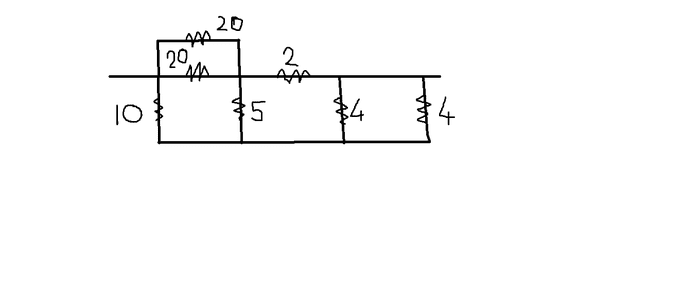Find the equivalent resistance between A and B. All resistances shown are in ohm.

## Homework Equations

The formulas for parallel and series connections.

## The Attempt at a Solution

I have attempted to redraw the circuit and my redrawn diagram looks like this.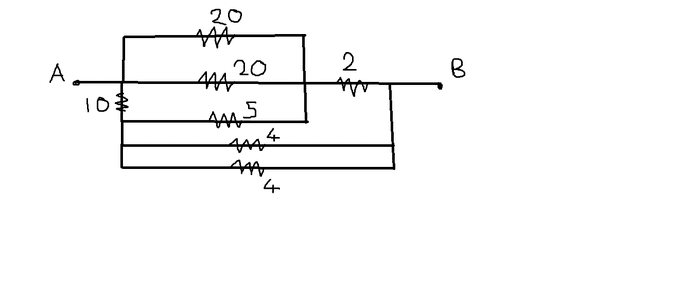I don't think it's correct,because I'm not getting the correct answer from this circuit diagram.
The only troublesome part is the 5 ohm resistor. My idea is that no current passes through it. If I make that assumption,then the answer comes out beautifully. Do I sniff a Wheatstone bridge (and zero potential difference) somewhere?

Looking at the original diagram, current can go through the first 4's then come back through the 5, This isn't possible on your diagram. (also it could then go through the 20 from the 5 - again, not possible on your diagram).

Not sure what the question is asking but I'd start simplifying resistance. So replace the first two 4s in parallel with a 2 ohm, and continue on in that fashion.

Word of warning. I'm horrible at these sort of things, I'm only here hoping someone can help me with one of my problems).

Current always takes the easiest path. Why would it be so keen to retrace its path and encounter a few extra resistances in the process?

Current always takes the easiest path. Why would it be so keen to retrace its path and encounter a few extra resistances in the process?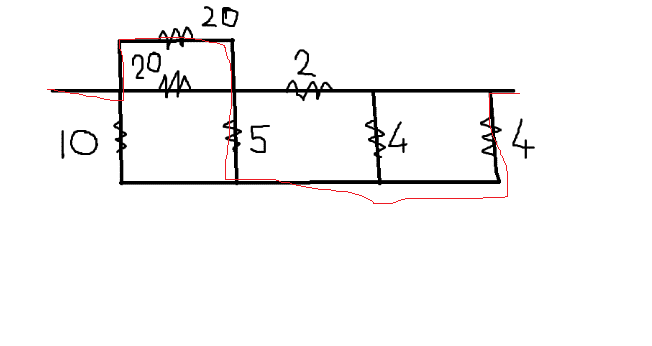Most of the current will take the easiest path, but that doesn't mean it all does.

Eg, Circuit has branch, one has 2ohm resistor, the other 4. Do you really think it ALL goes via the easier path?

Picture a supermarket queue. There's a fast moving 10 items or less queue, or a slow moving Checkout.
Sometimes you can stand behind 10 people going into the 10 items or less queue or you can wait for one person with a fat trolley of groceries.

If one of the paths were say 1000 Ohms then you can pretty much treat it as a broken wire, but otherwise, you have to consider all the options.

#### Attachments

I'd be thinking something like this :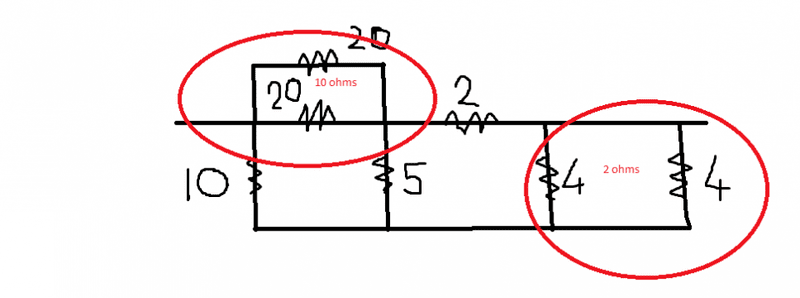And keep simplifying parallel circuits til things look simpler.

Again, warning, not my specialty, but something to think about.

You seem to have an answer, see if you can keep reducing it in that fashion and see what you get.

I actually calculated the net resistances for the original problem. Then I calculated the net resistance for the picture you drew, and I get the same net resistance in ohms. So I'd say you drew it correctly. Let me know if you need further explanation.

Here's my thinking, the two x 4s are essentially a single 2. So from that side it can go along either 2 ohm path. Then they'd cancel each other out going through the 5, so no current flows through that resistor.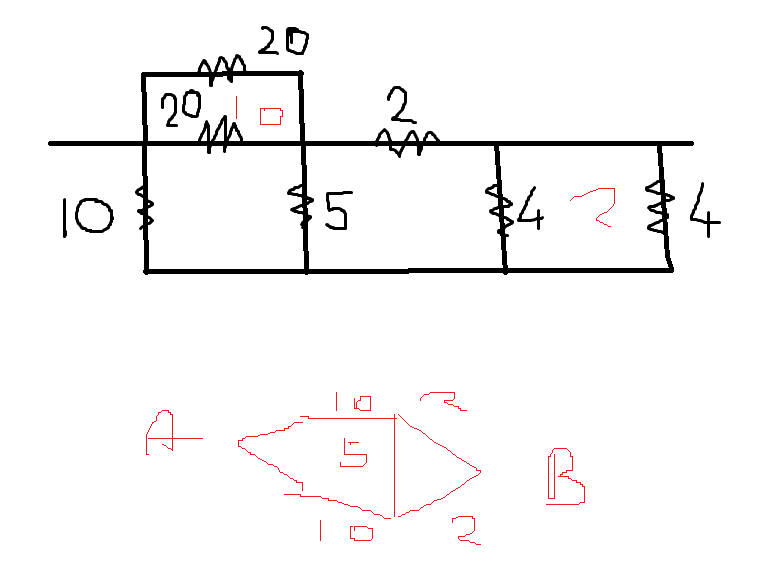•Arka420
mfb
Mentor
The transformed diagram in post #1 is fine, although more complicated than necessary. The approach of post 7 is easier, and it gives the right argument why no current flows through the 5 ohm resistor.

Here's my thinking, the two x 4s are essentially a single 2. So from that side it can go along either 2 ohm path. Then they'd cancel each other out going through the 5, so no current flows through that resistor.

View attachment 87088
Good thinking,man. I was thinking of coupling the two 20 ohm resistors,but I didn't think of coupling the two 4 ohm resistors. You did both,which revealed that the setup is nothing more than a simple Wheatstone bridge (a balanced one),which led to an easy answer (one without decimal digits).